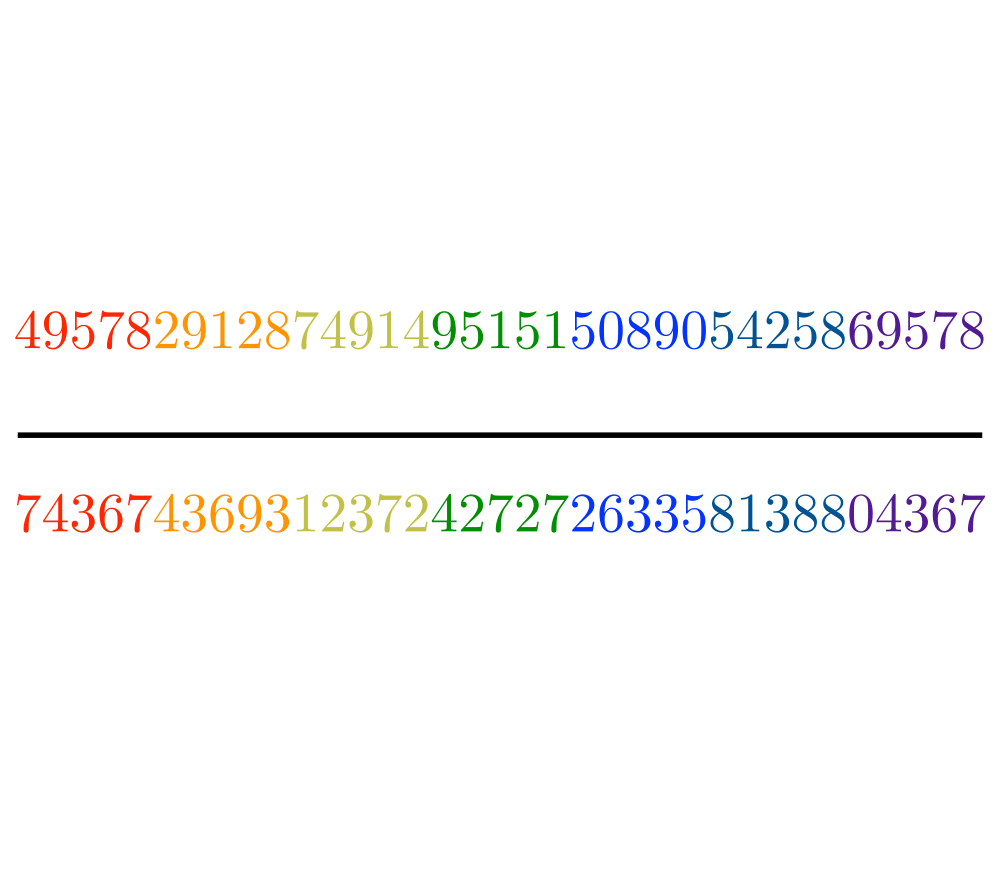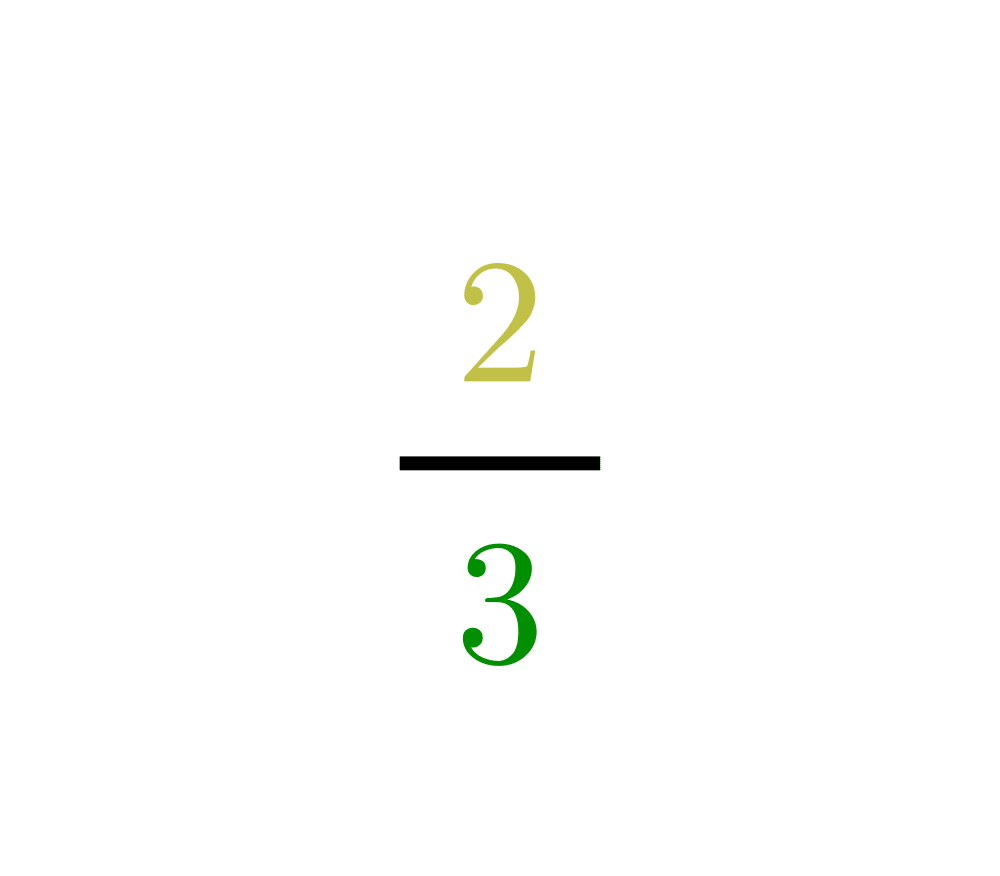# Arbitrary-Precision ArithmeticInputOutput

Input Description: Two very large integers, $$x$$ and $$y$$.
Problem: What is $$x+y$$, $$x-y$$, $$x x y$$ and $$x / y$$?

Excerpt from The Algorithm Design Manual: Any programming language whose level rises above basic assembler supports single- and perhaps double-precision integer/real addition, subtraction, multiplication, and division. But what if we wanted to represent the national debt of the United States in pennies? One trillion dollars worth of pennies requires 15 decimal digits, which is far more than can fit into a 32-bit integer.

In other applications much larger integers are needed. The RSA algorithm for public-key cryptography requires integer keys of at least 100 digits to achieve any level of security, and 1000 digits are recommended. Experimenting with number-theoretic conjectures for fun or research always requires playing with large numbers.

Go To Main Page